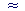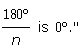11

# EVALUATING π

An inscribed polygon

The ratio of a chord to the diameter

The area of a circle

π IS THE RATIO of the circumference of a circle to the diameter. (Topic 9.)  But how shall we compare a curved line to a straight line? The answer is that we cannot do it directly. We can only relate straight lines to straight lines, and so we must approximate a curved line by a series of straight lines.  In this case, we approximate the circle by an inscribed polygon or a circumscribed polygon.The perimeter of the inscribed polygon will be less than the circumference of the circle, while the perimeter of the circumscribed polygon will be greater. We can then form the ratio of each perimeter to the diameter. That will produce a lesser and a greater approximation to π. Clearly, the more sides we take, the better the value.

An inscribed polygon

Each side of an inscribed polygon is a chord of the circle.  The perimeter of the polygon -- the approximation to the circumference -- will be the sum of all the chords.  From the following theorem we are able to evaluate π:

The ratio of a chord of a circle to the diameter
is given by the sine of half the central angle
that the chord subtends.We say that a chord subtends -- literally, stretches under -- a central angle.

Thus if AB is a chord of a circle, and CD the diameter, then

 ABCD =  sin θ2 .

Before proving this theorem, let us give some examples.

Example 1.   A chord subtends a central angle of 100°.  What ratio has the chord to the diameter?

Answer.  According to the theorem,

 Chord  Diameter =  sin ½(100°) Chord  Diameter =  sin 50° Chord  Diameter =  .766,   from the Table.

This means that the chord is 766 thousandths -- or a bit more than three fourths -- of the diameter.

Problem 1.   What ratio to the diameter has a chord that subtends a central angle of 60°?

To see the answer, pass your mouse over the colored area.
To cover the answer again, click "Refresh" ("Reload").

 Chord  Diameter =  sin ½(60°) = sin 30° = ½

This chord is half of the diameter.  This chord is equal to the radius!

Example 2.   A regular polygon of 8 sides is inscribed in a circle.  What central angle does each side subtend?  What ratio has each side to the diameter?  What ratio has the entire perimeter to the diameter?Answer.  Since the polygon has 8 sides, then each central angle is an eighth of the entire circle, that is, an eighth of 360°.   360° ÷ 8 = 45°.

Next,

 Chord  Diameter =  sin ½(45°) Chord  Diameter =  sin 22.5° Chord  Diameter.383,   from the Table.

As for the entire perimeter, it is made up of 8 such chords.  Therefore, the ratio of the perimeter to the diameter will be

8 × .383 = 3.064

This is an approximation to πFor,

 π = Circumference   DiameterPerimeterDiameter

The approximation is not a very good one, because we have approximated the circumference with a polygon of only 8 sides.

Problem 2.   Let a regular polygon of 20 sides be inscribed in a circle.

a)  Each side subtends what central angle?   360° ÷ 20 = 18°

b)  What ratio has each side to the diameter?  (Table)

 Chord  Diameter =  sin ½(18°) = sin 9° = .156
 c) What ratio has the entire perimeter to the diameter?  That is, approximate π.

The entire perimeter, is made up of 20 such chords. Therefore, the ratio of the perimeter to the diameter will be

20 × .156 = 3.12

We can generalize what we have done as follows.  Let us inscribe in a circle a polygon of n sides.  Then each side will subtend a central angle θ:

 θ  = 360°  n

Therefore,

 θ2 = 180°  n ,
 Chord  Diameter =  sin 180°  n

Finally, since n such chords approximates the circumference, then the ratio of those n chords to the diameter is an approximation to π:

 πn sin 180°  n

We shall use this below to prove that the area A of circle is

 A = π4 D2 .

Here is the proof of the ratio of a chord to the diameter.

Theorem. The ratio of a chord of a circle to the diameter is given by the sine of half the central angle that the chord subtends.Let E be the center of a circle with chord AB, diameter CD, and central angle AEB, which we will call θ; then

 ABCD = sin θ2 .

Draw EF so that it bisects angle θ.  Then EF is also the perpendicular bisector of AB,

because EA and EB are radii, and so triangle AEB is isosceles (Theorem 2).

Therefore,

 AF = ½ AB . And since EA is a radius, EA = ½ CD .Now,

 AFEA = sin θ2 . That is, ½ AB½ CD = sin θ2 . Therefore on multiplying both terms by 2, ABCD = sin θ2 . Chord  Diameter = sin θ2 .

This is what we set out to prove.

The area of a circle

In the previous Lesson, we saw how to know that area by the method of rearranging.  Here, we will prove the formula by means of inscribed polygons.

Theorem.  The area A of a circle is

 A = πr2  or π4 D2

where r is the radius of the circle, and D is the diameter.

Proof.  Let a regular polygon of n sides be inscribed in a circle of radius r, and let s be the length of each side.Let us divide the polygon into n isosceles triangles, and let us denote the area of each triangle by AT.  Then the area of those n triangles will approximate the area of the circle.

Now the area of each triangle is half the base s times the height h.

AT  =  ½sh .  .  .  .  .  .  .  .  .  (1)

We will now express both s and h in terms of the radius r, and then substitute those expressions in line (1).

 As we saw above, each chord s subtends a central angle of 360°  n .

Since the side of each isosceles triangle is the radius r, then h/r is the cosine of half that central angle:

 hr =  cos ½ 360°  n =  cos 180°  n

or,

 h  =  r cos 180°  n .  .  .  .  .  .  .  . (2)

Also, in each isosceles triangle,

 s/2  r =  sin 180°  n

so that

 s  =  2r sin 180°  n .  .  .  .  .  .  .  . (3)

Upon substituting lines (3) and (2) into line (1), we have

 AT = ½sh = ½· 2r sin 180°  n · r cos 180°  n
 AT = ½sh = r2 sin 180°  n · cos 180°  n

That is the area of one of the triangles.  The area A of the entire circle is approximated by all n triangles:

 A = nAT = n· r2 sin 180°  n · cos 180°  n

But we have seen that

 n sin 180°  nπ.

Therefore, finally,

 Aπr2 cos 180°  n .  .  .  .  .  .  .  . (4)

Suppose now that the number of sides n is an enormously large number -- more than the number of stars in a million galaxiesThen

 the polygon will "exhaust" the circle.   The central angle 180°  n (line 4)

will be indistinguishable from 0°.  We will have

A = πr2 cos 0°.

But cos 0° = 1.  Therefore,

A = πr2.

 Or, on replacing r2 with ( D2 )2 = D2 4 ,
 A = π4 D2.

This is what we wanted to prove.

When n is an extremely large number, then in the language of calculus, "The limit as n becomes infinite ofNext Topic:  Angles and their measurement